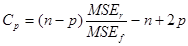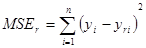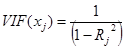U.S. Department of Transportation
1200 New Jersey Avenue, SE
Washington, DC 20590
202-366-4000

Federal Highway Administration Research and Technology
Coordinating, Developing, and Delivering Highway Transportation Innovations

 REPORT This report is an archived publication and may contain dated technical, contact, and link information
 Publication Number:  FHWA-HRT-12-030    Date:  August 2012
 Publication Number: FHWA-HRT-12-030 Date: August 2012

# Estimation of Key PCC, Base, Subbase, and Pavement Engineering Properties From Routine Tests and Physical Characteristics

PDF files can be viewed with the Acrobat® Reader®

# CHAPTER 5. MODEL DEVELOPMENT (2)

### Statistical Criteria for Used for Model Development

The statistical analyses performed in this study examined several statistical parameters in choosing the optimal model and in determining the accuracy of the model. The process included evaluating various aspects of the model, and the following parameters were generally verified:

• Cp—A statistical term to select the best subset of regressors for a model and an indicator of the collinearity of a regression model.
• VIF—A statistical term to evaluate the multicollinearity of the model (i.e., it tracks the interaction effects of the regressors identified).
• p-value—A probability calculation to ascertain the significance of the regressor in the equation.
• R2—A statistic that indicates the goodness of fit of a model and describes how closely the regression line fits the data points.

### Cp

Mallows’ Cp is often used as the criterion for selecting the most appropriate sub-model of p regressors (or independent variables) from a full model of k regressors, p < k.(143) In the current study, the potential variables that could likely influence the value of the dependent variable were identified from a literature review of specific material parameters. However, it is not clear whether the specific dataset being used to develop the models can suitably show the correlation expected. In other words, the initial attempt in developing the model could likely include more variables or regressors than the model can handle. This can result in forcing variables that are highly correlated and whose effects cannot be independently estimated or isolated by the model. The Cp term that is used in a step-wise regression process helps avoid an over-fit model by identifying the best subset of only the important predictors of the dependent variable.

Cp takes into account the mean square error for the two models and the number of variables in the reduced model as seen in figure 125.#### Figure 125. Equation. Cp.

Where:

n = The sample size. MSEr = The mean square error for the regression for the smaller model of p regressors and is expressed as follows:#### Figure 126. Equation. MSEr.

MSEf is the mean square error for the regression on the full model of k regressors. Note that for p = k, MSEr = MSEf and Cp = p.

Sub-models are ordered in SAS® based on Cp; the smaller the Cp value, the better. While it is a reliable measure of the goodness of fit for a model, it is fairly independent of R2 in determining the number of predictors in the model. SAS® also lists R2 for each model created with data subsets, which greatly enables the selection of a feasible submodel for further evaluation. However, the variables in the reduced model must all be significantly different from zero and cannot be too correlated, which is verified using VIF.

### VIF

Generally, VIF can be regarded as the inverse of tolerance. The square root of VIF indicates how much larger the standard error is compared with what it would be if that variable is uncorrelated with the other independent variables in the equation.

If y is regressed on a set of x variables x1 to xk, VIFs of all x variables should be created in the following manner:

For variable xj, VIF is the inverse of (1 - R2) from the regression of xj on the remainder of the x variables. In other words, xj regressed on x1xj - 1, xj+1xk, produces a regression with R2 as Rj2. Therefore, figure 127 was created as follows:#### Figure 127. Equation. VIF.

VIF is always greater than 1. A VIF value of 10 indicates that 90 percent of xj is not explained by the other x variables. A common rule of thumb is that if VIF for any variable is greater than 5, multicollinearity exists for that variable and should be excluded from the model. However, in cases where the parameter is either known to correlate well or other variables do not provide a reasonable model, a cut-off value of 10 is acceptable but less preferred.

### R2

R2 is the coefficient of determination and is the square of the sample correlation coefficient computed between the outcomes and their predicted values, or, in the case of simple linear regression, between the outcome and the values being used for prediction. R2 values vary from zero to 1 and are expressed as a percentage. An R2 of x percent indicates x percent of the variation in the response variable can be explained by the explanatory variable, and (100 - x) percent can be explained by unknown variability. The higher the value of this term, the greater the predictive ability of the model. It is the most commonly used statistic to evaluate the quality of fit achieved with a model.

From the standpoint of using R2 to select a model, while relationships with higher values are desirable, it is not to be treated as the ultimate criterion to establish the model. R2 needs to be interpreted with reasonable caution and needs to be combined with the information from the other statistical parameters discussed in this section. In fact, it is not the first check to select a model; instead, it should serve as the final check to establish the model.

The statistical parameters discussed previously do not individually optimize a model; instead, these parameters need to be evaluated in combination to derive the most accurate model. Furthermore, it is imperative in establishing a model that both statistical and engineering aspects be balanced. The accuracy of the model needs to be verified for technical/engineering validity by evaluating each variable in the model and confirming that the observed trends are as expected (verified in literature) and that the effect of the independent variable on the predicted variable is reasonable (verified through sensitivity analyses).

The following list describes the limitations of the Cp, VIF, and R2 parameters and the methods used to overcome them:

• Cp, VIF, and R2 do not explain whether the independent variables are a true cause of the changes in the dependent variable or if the trends predicted by the model are accurate. In other words, they do not identify a random correlation between two variables. Engineering knowledge (or knowledge from outside of the modeling exercise) needs to be incorporated in accepting or rejecting regressors or a model form.
• Cp, VIF, and R2 do not explain whether the model has a bias because of a variable that has been omitted from the list of regressors. As a result, it is important to include, within practical considerations, all variables even remotely known to affect the predicted parameter in the model for a preliminary analysis to determine if the variables are correlated.
• R2 does not indicate whether the variables included in the model are significant. The p-value should be limited to the level of confidence desired in the model. Typically, a confidence level of 95 percent is used, such that the values should remain below 0.05. However, in rare cases, this sometimes is limited to 0.1.
• R2 alone does not indicate whether there is collinearity present in the data or whether the selected independent variables have an interaction effect. It is necessary to verify using Cp and VIF.
• Cp, VIF, and R2 do not offer any suggestions regarding further scope to improve a model by using transformed versions of the existing set of independent variables. Again, the use of engineering knowledge is necessary to incorporate transformed variables.

## Other Modeling Considerations

### Interaction Effects of Independent Parameters

Information from the literature points to the influence of independent variables on each material property of interest (the dependent variables) in a general sense, without adequately accounting for the impact other design and site parameters or independent variables may have on the dependent parameters. Therefore, to draw consistent and dependable conclusions on the effect of each independent parameter, it would be ideal to compare scenarios that have all other variables constant or in common, except for the independent variable under consideration, such as the effect of w/c ratio on strength or base type on erosion.

However, in synthesizing information from large databases, as was done in the present study, it is essential to adopt statistical tools to assess the relationships between several independent variables and the dependent variable. Therefore, where necessary, both linear regressions and the generalized linear model (GLM) were utilized to establish a model. GLM can independently examine the influence of an independent variable on a dependent variable despite the presence of other predictor variables in the data sample. In other words, GLM can isolate the effects of one independent variable by normalizing the effect of others, and it predicts whether the effect of each independent variable is statistically significant on a dependent variable using the analysis of variance (ANOVA) method.

GLM is a generalization of the linear regression model and can accommodate the following:

• Non-linear and linear effects of independent variables.
• Categorical predictor variables as well as continuous predictor variables.
• Dependent variables whose distributions follow several special members of the exponential family of distributions (e.g., gamma, Poisson, binomial, etc.), as well as normally distributed dependent variable.

### Multilevel ANOVA Models

Multilevel ANOVA models are more complex models used in the design of experiments, and in the context of the current study, they are more appropriate to use when the dataset contains multiple measures or clustered tests. The analyses should account for the fact that the other regressors in the equation are the same for multiple levels of one of the parameters, which most often is the pavement age parameter in the current study. This also is called a hierarchical model.

An example of such a model is one that compares PCC compressive strength for core and cylinder measurements. The LTPP database contains compressive strength results for cylinders cast during construction and cores taken from the pavement for SPS sections. These cores and cylinders have been tested at 14 days, 28 days, and 1 year. The strengths can be compared for each section and age. A simple way of doing such a comparison would be to perform a paired t-test. However, the number of measurements due to repeated measurements at different ages (i.e., 14 days, 28 days, 1 year, 2 years, etc.) should not be allowed to count as a full data point for sections with more than one age measurement. Therefore, a multilevel ANOVA model featuring State and sections should be used. If the data are balanced so that there are the same number of observations for each age and section, the paired t-test and the multilevel ANOVA would show the same results in the test whether core and cylinder measurements differ. In this example, the dataset is not balanced so the tests are not the same, with the multilevel ANOVA being the more appropriate analysis. Likewise, while developing a model to estimate strength at any age, the age parameter has to be treated in a hierarchical fashion.

All observations have the same fabrication variables at the State by section code level, and these are repeated when sections are tested several times (i.e., at different ages). It is not appropriate that the design values for a section tested four times should be allowed to count four times. Therefore, a multilevel ANOVA model must be used to guarantee that values from each section count only once while the values measured over time are incorporated in the analysis.

### Treatment of Outliers

Generally, a true model representing the dataset used should include all natural data in the dataset. In other words, deliberate changes or removal of data artificially alters the inherent model. However, in using large datasets, especially when field data are used or when the data are from a dataset not originally designed to develop the model, values that lie beyond the scope of a field's value range are encountered. Such data, referred to as outliers, cannot be explained by other parameters specific to that case or observation. In statistical models, outliers are given special consideration and treated in a consistent manner for all points in the model so as to not simulate a fabricated dataset.

Outliers are either deleted (treated as missing values) or capped at a minimum or maximum value for each variable. In the current study, to the extent possible, outliers were not deleted from the datasets. However, certain models necessitated the deletion of select data points. When outliers were deleted, the process was based on a consistent criterion. Treatment of outliers is discussed separately for each model.

### Grouping of Datasets

Any grouping of datasets performed is discussed separately for each model.

Federal Highway Administration | 1200 New Jersey Avenue, SE | Washington, DC 20590 | 202-366-4000
Turner-Fairbank Highway Research Center | 6300 Georgetown Pike | McLean, VA | 22101